monte_carlo_model_free_prediction_and_control slides

## Model Free Prediction¶

Draft status

### Monte Carlo Methods¶

#### Monte Carlo Policy Evaluation¶

• Goal: learn $v_\pi$ from episodes of experience under policy $\pi$
• $S_1, A_1, R_2, \dots, S_T \sim \pi$
• The return is the total discounted reward:
• $G_t = R_{t+1} + \gamma R_{t+2} + \gamma^2 R_{t+2} + \dots$
• The value function is the expected return:
• $v_\pi (s) = \mathbb E_\pi[G_t \mid S_t=s]$

Monte-Carlo policy evaluation uses empirical mean return instead of expected return.

#### First-Vistit Monte-Carlo Policy Evaluation¶

• to evaluate state $s$
• the first time $t$ the state $s$ is visited in an episode,
• increment counter: $N(s) \leftarrow N(s) +1$
• increment total return: $S(s) \leftarrow S(s) + G_t$
• value is estimated by mean return $v(s) = S(s)/N(s)$
• by law of large numbers, $V(s) \rightarrow v_\pi(s)$ as $N(s) \rightarrow \infty$

#### Every-Vistit Monte-Carlo Policy Evaluation¶

• to evaluate state $s$
• every time $t$ the state $s$ is visited in an episode,
• increment counter: $N(s) \leftarrow N(s) +1$
• increment total return: $S(s) \leftarrow S(s) + G_t$
• value is estimated by mean return $V(s) = S(s)/N(s)$
• by law of large numbers, $V(s) \rightarrow v_\pi(s)$ as $N(s) \rightarrow \infty$

### OpenAI Gyms "Black Jack" environment¶

In :
import numpy as np

In :
import gym
env = gym.make('Blackjack-v0')
env.action_space

[2017-05-22 15:01:13,988] Making new env: Blackjack-v0

Out:
Discrete(2)

There are two actions:

• 0: stay
• 1: hit
In :
#random action
env.action_space.sample()

Out:
1
In :
help(env)

Help on BlackjackEnv in module gym.envs.toy_text.blackjack object:

class BlackjackEnv(gym.core.Env)
|  Simple blackjack environment
|
|  Blackjack is a card game where the goal is to obtain cards that sum to as
|  near as possible to 21 without going over.  They're playing against a fixed
|  dealer.
|  Face cards (Jack, Queen, King) have point value 10.
|  Aces can either count as 11 or 1, and it's called 'usable' at 11.
|  This game is placed with an infinite deck (or with replacement).
|  The game starts with each (player and dealer) having one face up and one
|  face down card.
|
|  The player can request additional cards (hit=1) until they decide to stop
|  (stick=0) or exceed 21 (bust).
|
|  After the player sticks, the dealer reveals their facedown card, and draws
|  until their sum is 17 or greater.  If the dealer goes bust the player wins.
|
|  If neither player nor dealer busts, the outcome (win, lose, draw) is
|  decided by whose sum is closer to 21.  The reward for winning is +1,
|  drawing is 0, and losing is -1.
|
|  The observation of a 3-tuple of: the players current sum,
|  the dealer's one showing card (1-10 where 1 is ace),
|  and whether or not the player holds a usable ace (0 or 1).
|
|  This environment corresponds to the version of the blackjack problem
|  described in Example 5.1 in Reinforcement Learning: An Introduction
|  by Sutton and Barto (1998).
|  https://webdocs.cs.ualberta.ca/~sutton/book/the-book.html
|
|  Method resolution order:
|      BlackjackEnv
|      gym.core.Env
|      builtins.object
|
|  Methods defined here:
|
|  __init__(self, natural=False)
|      Initialize self.  See help(type(self)) for accurate signature.
|
|  ----------------------------------------------------------------------
|  Methods inherited from gym.core.Env:
|
|  __del__(self)
|
|  __str__(self)
|      Return str(self).
|
|  close(self)
|      Override _close in your subclass to perform any necessary cleanup.
|
|      Environments will automatically close() themselves when
|      garbage collected or when the program exits.
|
|  configure(self, *args, **kwargs)
|
|  render(self, mode='human', close=False)
|      Renders the environment.
|
|      The set of supported modes varies per environment. (And some
|      environments do not support rendering at all.) By convention,
|      if mode is:
|
|      - human: render to the current display or terminal and
|        return nothing. Usually for human consumption.
|      - rgb_array: Return an numpy.ndarray with shape (x, y, 3),
|        representing RGB values for an x-by-y pixel image, suitable
|        for turning into a video.
|      - ansi: Return a string (str) or StringIO.StringIO containing a
|        terminal-style text representation. The text can include newlines
|        and ANSI escape sequences (e.g. for colors).
|
|      Note:
|            the list of supported modes. It's recommended to call super()
|            in implementations to use the functionality of this method.
|
|      Args:
|          mode (str): the mode to render with
|          close (bool): close all open renderings
|
|      Example:
|
|      class MyEnv(Env):
|          metadata = {'render.modes': ['human', 'rgb_array']}
|
|          def render(self, mode='human'):
|              if mode == 'rgb_array':
|                  return np.array(...) # return RGB frame suitable for video
|              elif mode is 'human':
|                  ... # pop up a window and render
|              else:
|                  super(MyEnv, self).render(mode=mode) # just raise an exception
|
|  reset(self)
|      Resets the state of the environment and returns an initial observation.
|
|      Returns: observation (object): the initial observation of the
|          space.
|
|  seed(self, seed=None)
|      Sets the seed for this env's random number generator(s).
|
|      Note:
|          Some environments use multiple pseudorandom number generators.
|          We want to capture all such seeds used in order to ensure that
|          there aren't accidental correlations between multiple generators.
|
|      Returns:
|          list<bigint>: Returns the list of seeds used in this env's random
|            number generators. The first value in the list should be the
|            "main" seed, or the value which a reproducer should pass to
|            'seed'. Often, the main seed equals the provided 'seed', but
|            this won't be true if seed=None, for example.
|
|  step(self, action)
|      Run one timestep of the environment's dynamics. When end of
|      episode is reached, you are responsible for calling reset()
|      to reset this environment's state.
|
|      Accepts an action and returns a tuple (observation, reward, done, info).
|
|      Args:
|          action (object): an action provided by the environment
|
|      Returns:
|          observation (object): agent's observation of the current environment
|          reward (float) : amount of reward returned after previous action
|          done (boolean): whether the episode has ended, in which case further step() calls will return undefined results
|          info (dict): contains auxiliary diagnostic information (helpful for debugging, and sometimes learning)
|
|  ----------------------------------------------------------------------
|  Static methods inherited from gym.core.Env:
|
|  __new__(cls, *args, **kwargs)
|      Create and return a new object.  See help(type) for accurate signature.
|
|  ----------------------------------------------------------------------
|  Data descriptors inherited from gym.core.Env:
|
|  __dict__
|      dictionary for instance variables (if defined)
|
|  __weakref__
|      list of weak references to the object (if defined)
|
|  monitor
|
|  unwrapped
|      Completely unwrap this env.
|
|      Returns:
|          gym.Env: The base non-wrapped gym.Env instance
|
|  ----------------------------------------------------------------------
|  Data and other attributes inherited from gym.core.Env:
|
|  action_space = None
|
|
|  observation_space = None
|
|  reward_range = (-inf, inf)


In :
observation_space = env.observation_space.spaces
observation_space

Out:
(Discrete(32), Discrete(11), Discrete(2))

Observation tuple for Blackjack:

• sum of players cards (ace counts 11)
• sum of dealers cards
• player has useable ace
In :
# Resets the state of the environment and returns an initial observation.
observation = env.reset()
observation

Out:
(14, 6, False)
In :
# env.step:
# Run one timestep of the environment's dynamics. When end of
# episode is reached, you are responsible for calling reset()
# to reset this environment's state.
action = 0
observation, reward, done, info = env.step(action)
observation, reward, done, info

Out:
((14, 6, False), 1.0, True, {})

#### Policy¶

For each observation we need an action:

In :
# There are invalid states, but we don't care for convenience.
state_space_size = (33, 12, 2)

In :
# simple policy:
policy = np.zeros(state_space_size, dtype=int)
#policy[:9,:,:]=1

In :
def observation_clean(observation):
return (observation, observation, int(observation))

observation = observation_clean(observation)
policy[observation]

Out:
0
In :
policy[observation]

Out:
0
In :
np.random.rand()

Out:
0.6780772481806671

### Monte Carlo Policy Evaluation¶

• Policy Evaluation
• Policy Improvement
• via $\pi(s) = \text{arg} \max_a q(s,a)$

Note: That the states are different in the OpenAI gym (here) and in [SuBa]:

• here an ace counts always 11 for the player. But dealer showing '1' means an ace.
• in [SuBa] only if there is no useable ace.
In :
def run_episode(policy, env=env):
steps = []
observation = observation_clean(env.reset())
done = False
steps.append(((None, None)+ (observation, 0)))
while not done:
action = policy[observation]
observation_action = (observation, action)
observation, reward, done, info = env.step(action)
observation = observation_clean(observation)
steps.append(observation_action + (observation, int(reward)))
return steps # list of tupels: (s, a, s', R)

In :
run_episode(policy)

Out:
[(None, None, (13, 10, 1), 0), ((13, 10, 1), 0, (13, 10, 1), 1)]
In :
gamma = 0.99

In :
N = np.zeros(state_space_size, dtype=int)
S = np.zeros(state_space_size)

# every visit monte carlo:
nb_of_episodes = 100000
for e in range(nb_of_episodes):
observations_reward = run_episode(policy)
G = 0.
#print (observations_reward )
for o0, a, o, r in reversed(observations_reward):
G = r + gamma * G
N[o] += 1
S[o] += G
#print (o,r,G)

In :
Gs = np.zeros(state_space_size)
Gs[N!=0] = S[N!=0]/N[N!=0]

In :
import matplotlib.pyplot as plt
from mpl_toolkits.mplot3d import Axes3D
from matplotlib import cm
%matplotlib inline

In :
Gs[10, 3, 1]

Out:
0.0
In :
A = np.arange(1, 11)
B = np.arange(4, 22)
A, B = np.meshgrid(A, B)

V = Gs[4:22, 1:11, 0]
fig = plt.figure(figsize=(10,8))
ax = fig.gca(projection='3d')
surf = ax.plot_surface(A, B, V, rstride=1, cstride=1, cmap=cm.coolwarm,
linewidth=0, antialiased=False)
ax.set_ylabel("Player sum")
ax.set_xlabel("Dealer showing")
ax.set_title("No useable Ace")
fig.colorbar(surf, shrink=0.5, aspect=5)
plt.show()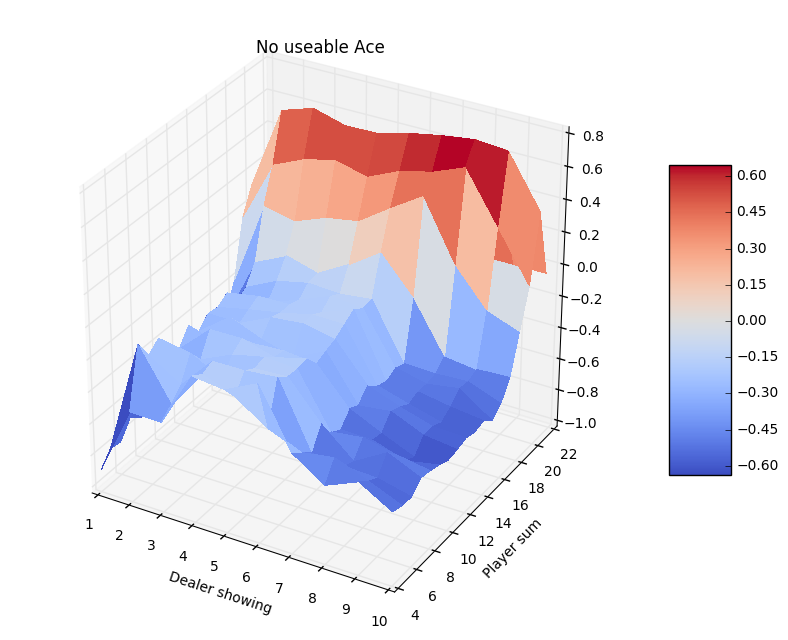In :
A = np.arange(1, 11)
B = np.arange(12, 22)
A, B = np.meshgrid(A, B)

V = Gs[12:22, 1:11, 1]
fig = plt.figure(figsize=(10,8))
ax = fig.gca(projection='3d')
surf = ax.plot_surface(A, B, V, rstride=1, cstride=1, cmap=cm.coolwarm,
linewidth=0, antialiased=False)
ax.set_ylabel("Player sum")
ax.set_xlabel("Dealer showing")
ax.set_title("Useable Ace")
fig.colorbar(surf, shrink=0.5, aspect=5)
plt.show()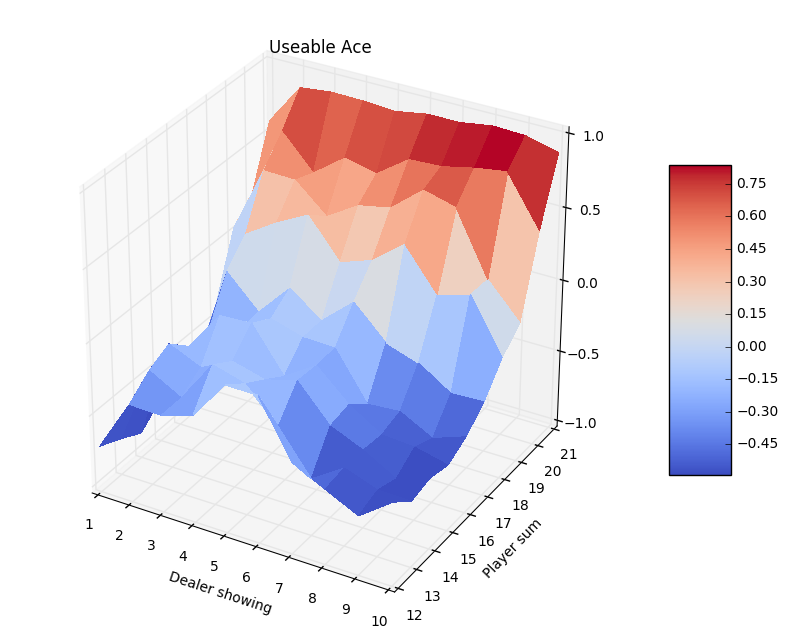#### Exercises¶

• Try Monte Carlo Policy Evaluation with different number of epochs to get a feeling of the convergence properties. Take a look at the 3d-plots.

### Monte Carlo Control¶

Two assumptions for finding optimal policy:

For Black Jack the "exploring starts"-condition can be satisfied, if we choose the first action randomly. So let's modify the run_episode-function:

In :
def run_episode_exploring_start(policy, env=env):
steps = []
observation = observation_clean(env.reset())
done = False
steps.append(((None, None)+ (observation, 0)))
start = True
while not done:
if start:
action = np.random.binomial(n=1, p=0.5)
start = False
else:
action = policy[observation]
observation_action = (observation, action)
observation, reward, done, info = env.step(action)
observation = observation_clean(observation)
steps.append(observation_action + (observation, int(reward)))
return steps # list of tupels: (s, a, s', R)

In :
size = list(state_space_size) + 
Q = np.zeros(size)
Q.shape

Out:
(33, 12, 2, 2)
In :
from collections import defaultdict
returns = defaultdict(list)

In :
nb_of_episodes = 500000

def monte_carlo_optimal_policy(nb_of_episodes,
policy = np.random.binomial(n=1, p=0.5, size=state_space_size),
run_episode = run_episode_exploring_start):

for i in range(nb_of_episodes):
# (a) generate an episode using exploring starts and pi
observations_reward = run_episode(policy)

G = 0. # current return
# use a map for the first visit condition:
o_a = {} # map from states to (action, return)-Tuple
for o0, a, o, r in reversed(observations_reward):
G = r + gamma * G
o_a[o0] = a, G

#(b) for each pair (s,a) appearing in the episode
for o, (a, G) in o_a.items():
if o is not None:
returns[(o, a)].append(G)
re_mean = np.array(returns[(o, a)]).mean()
Q[(o) + (a,)] = re_mean

# for each s in the episode: optimize policy
policy[o] = np.argmax(Q[(o)])
return policy

policy = monte_carlo_optimal_policy(nb_of_episodes)

In :
Q[5, 8, 0, 1]

Out:
-0.15549859009756098
In :
policy[5,5,0]

Out:
1
In :
import scipy.ndimage

def plot_policy_useable_ace(policy):
B = np.arange(4-0.5, 22-0.5, 0.2)
A = np.arange(1-0.5, 11-0.5, 0.2)
A, B = np.meshgrid(A, B)

Po = scipy.ndimage.zoom(policy[4:22, 1:11, 0], 5)

levels = range(-1,2)
plt.figure(figsize=(7,6))
CS = plt.contourf(A, B, Po, levels)
cbar = plt.colorbar(CS)
cbar.ax.set_ylabel('actions')
#plt.clabel(CS, inline=1, fontsize=10)
plt.title('Policy for no useable ace')
plt.xlabel("dealers showing")
plt.ylabel("Players sum")
_ = plt.xticks(range(1,11))
_ = plt.yticks(range(4,22))

In :
# TODO improve plots
plot_policy_useable_ace(policy)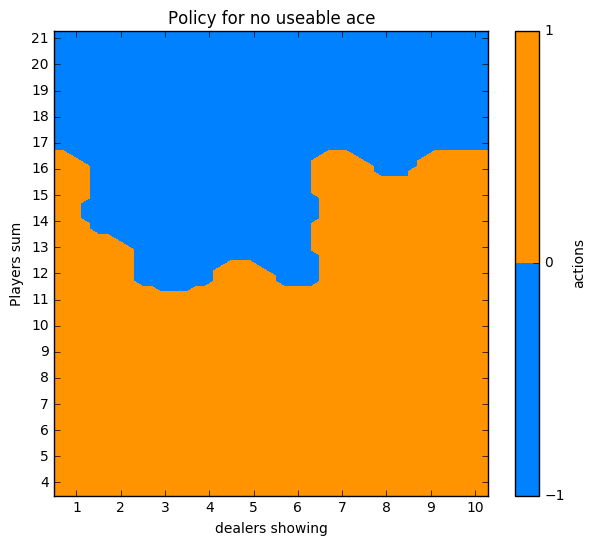In :
def plot_policy_no_useable_ace(policy):
B = np.arange(12-0.5, 22-0.5, 0.2)
A = np.arange(1-0.5, 11-0.5, 0.2)
A, B = np.meshgrid(A, B)

Po = scipy.ndimage.zoom(policy[12:22, 1:11, 1], 5, mode='nearest')

levels = range(-1,2)
plt.figure(figsize=(7,6))
CS = plt.contourf(A, B, Po, levels)
cbar = plt.colorbar(CS)
cbar.ax.set_ylabel('actions')
#plt.clabel(CS, inline=1, fontsize=10)
plt.title('Policy for useable ace')
plt.xlabel("dealers showing")
plt.ylabel("Players sum")
_ = plt.xticks(range(1,11))
_ = plt.yticks(range(12,22))

In :
plot_policy_no_useable_ace(policy)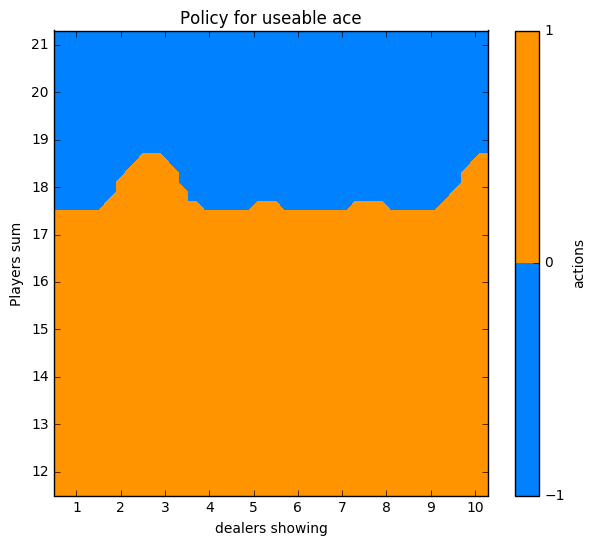#### On-policy Monte Carlo Control¶

If the exploring starts condition can not be satisfied, because not every state $s \in \mathcal S$ can be a start state, we need to have an other strategy for exploration.

A simple method is $\epsilon$-greedy, i.e. with probability $\epsilon$ we choose a random action. So the policy we are following is non-deterministic: $\pi(a \mid s)$.

In the implementation the policy of the array policy is chosen with probability $1-\epsilon/2$ and the alternative action with probability $\epsilon/2$.
Note that for each state $s \in \mathcal S$: $|\mathcal A(s)| = 2$.

Note that the final policy is not the deterministic policy stored in policy. It's a soft $\epsilon$-greedy policy: The actions should be taken from policy with probabiliy $1-\epsilon/2$ and the alternative action with probability $\epsilon /2$.

In :
def run_episode_epsilon_greedy(policy, epsilon=0.05, env=env):
steps = []
observation = observation_clean(env.reset())
done = False
steps.append(((None, None)+ (observation, 0)))
while not done:
if np.random.rand() > epsilon:
action = policy[observation]
else:
action = np.random.binomial(n=1, p=0.5)
observation_action = (observation, action)
observation, reward, done, info = env.step(action)
observation = observation_clean(observation)
steps.append(observation_action + (observation, int(reward)))
return steps # list of tupels: (s, a, s', R)

In :
policy = monte_carlo_optimal_policy(nb_of_episodes, run_episode=run_episode_epsilon_greedy)

In :
# action taken with probabilty: 1 - epsilon/2
plot_policy_useable_ace(policy)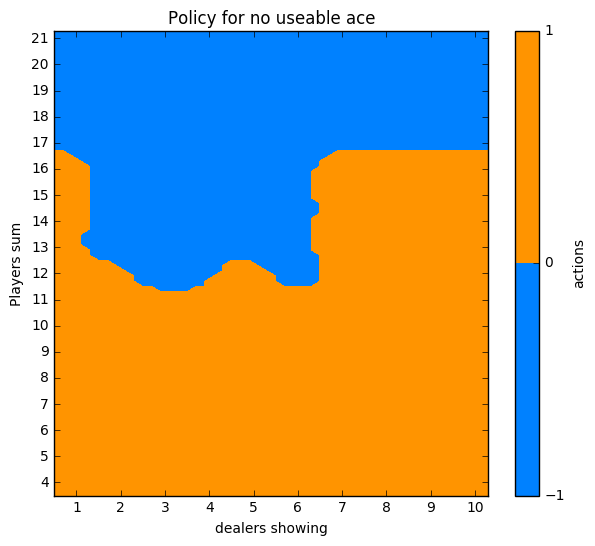In :
#action taken with probabilty: 1 - epsilon/2
plot_policy_no_useable_ace(policy)### Off-Policy Evaluation¶

Goal:

• following a policy $\pi'$ (behavior policy)
• estimate for a different policy $\pi$: $v^\pi$ resp. $q^\pi$ (estimation policy)
• $\pi \neq \pi'$

require:

• if $\pi( a \mid s)>0$ then $\pi'( a \mid s)>0$, so we have episodes with action $a$ taken in states $s$.

Idea: "Importance sampling of the whole episodes", see [SuBa] 5.5 Evaluating One Policy While Following Another

So the $\epsilon$-greedy (estimation policy) policy can be evaluated and optimized while following the soft $\epsilon$-greedy policy, see [SuBa] 5.6 Off-Policy Monte Carlo Control.

• Update $v(s)$ incrementally after episode $S_1, A_1, R_2, \dots, S_T$
• For each state $S_t$ with return $G_t$
• $N(S_t) \leftarrow N(S_t) +1$
• $V(S_t) \leftarrow V(S_t) + \frac{1}{N(S_t)} (G_t - V(S_t))$
• In non-stationary problems, it can be useful to track a running mean, i.e. forget old episodes:
• $V(S_t) \leftarrow V(S_t) + \alpha (G_t - V(S_t))$

#### Incremental mean¶

$$\mu_k = \frac{1}{k}\sum_{j=1}^k x_j = \frac{1}{k} \left( x_k + \sum_{j=1}^{k-1} x_j \right) =\frac{1}{k} \left( x_k + (k+1) \mu_{k-1}\right) = \mu_{k-1} + \frac{1}{k} (x_k - \mu_{k-1})$$

#### Exercise¶

• Modify the code for first-visit MC policy evaluation and control to use the incremental implementation.